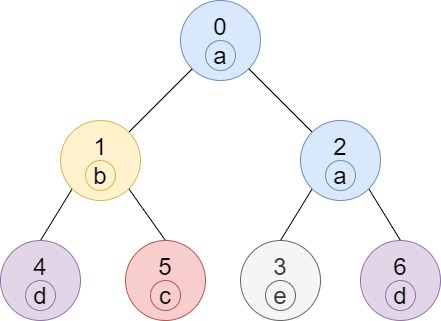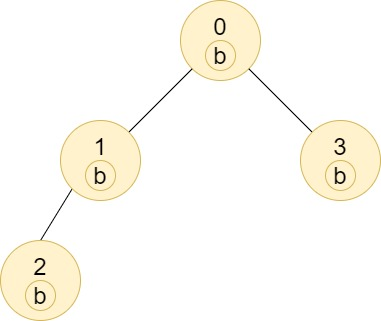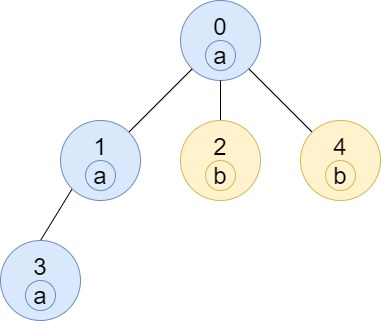# GeetCode Hub

Given a tree (i.e. a connected, undirected graph that has no cycles) consisting of `n` nodes numbered from `0` to `n - 1` and exactly `n - 1` `edges`. The root of the tree is the node `0`, and each node of the tree has a label which is a lower-case character given in the string `labels` (i.e. The node with the number `i` has the label `labels[i]`).

The `edges` array is given on the form `edges[i] = [ai, bi]`, which means there is an edge between nodes `ai` and `bi` in the tree.

Return an array of size `n` where `ans[i]` is the number of nodes in the subtree of the `ith` node which have the same label as node `i`.

A subtree of a tree `T` is the tree consisting of a node in `T` and all of its descendant nodes.

Example 1:```Input: n = 7, edges = [[0,1],[0,2],[1,4],[1,5],[2,3],[2,6]], labels = "abaedcd"
Output: [2,1,1,1,1,1,1]
Explanation: Node 0 has label 'a' and its sub-tree has node 2 with label 'a' as well, thus the answer is 2. Notice that any node is part of its sub-tree.
Node 1 has a label 'b'. The sub-tree of node 1 contains nodes 1,4 and 5, as nodes 4 and 5 have different labels than node 1, the answer is just 1 (the node itself).
```

Example 2:```Input: n = 4, edges = [[0,1],[1,2],[0,3]], labels = "bbbb"
Output: [4,2,1,1]
Explanation: The sub-tree of node 2 contains only node 2, so the answer is 1.
The sub-tree of node 3 contains only node 3, so the answer is 1.
The sub-tree of node 1 contains nodes 1 and 2, both have label 'b', thus the answer is 2.
The sub-tree of node 0 contains nodes 0, 1, 2 and 3, all with label 'b', thus the answer is 4.
```

Example 3:```Input: n = 5, edges = [[0,1],[0,2],[1,3],[0,4]], labels = "aabab"
Output: [3,2,1,1,1]
```

Example 4:

```Input: n = 6, edges = [[0,1],[0,2],[1,3],[3,4],[4,5]], labels = "cbabaa"
Output: [1,2,1,1,2,1]
```

Example 5:

```Input: n = 7, edges = [[0,1],[1,2],[2,3],[3,4],[4,5],[5,6]], labels = "aaabaaa"
Output: [6,5,4,1,3,2,1]
```

Constraints:

• `1 <= n <= 10^5`
• `edges.length == n - 1`
• `edges[i].length == 2`
• `0 <= ai, bi < n`
• `ai != bi`
• `labels.length == n`
• `labels` is consisting of only of lower-case English letters.

class Solution { public int[] countSubTrees(int n, int[][] edges, String labels) { } }